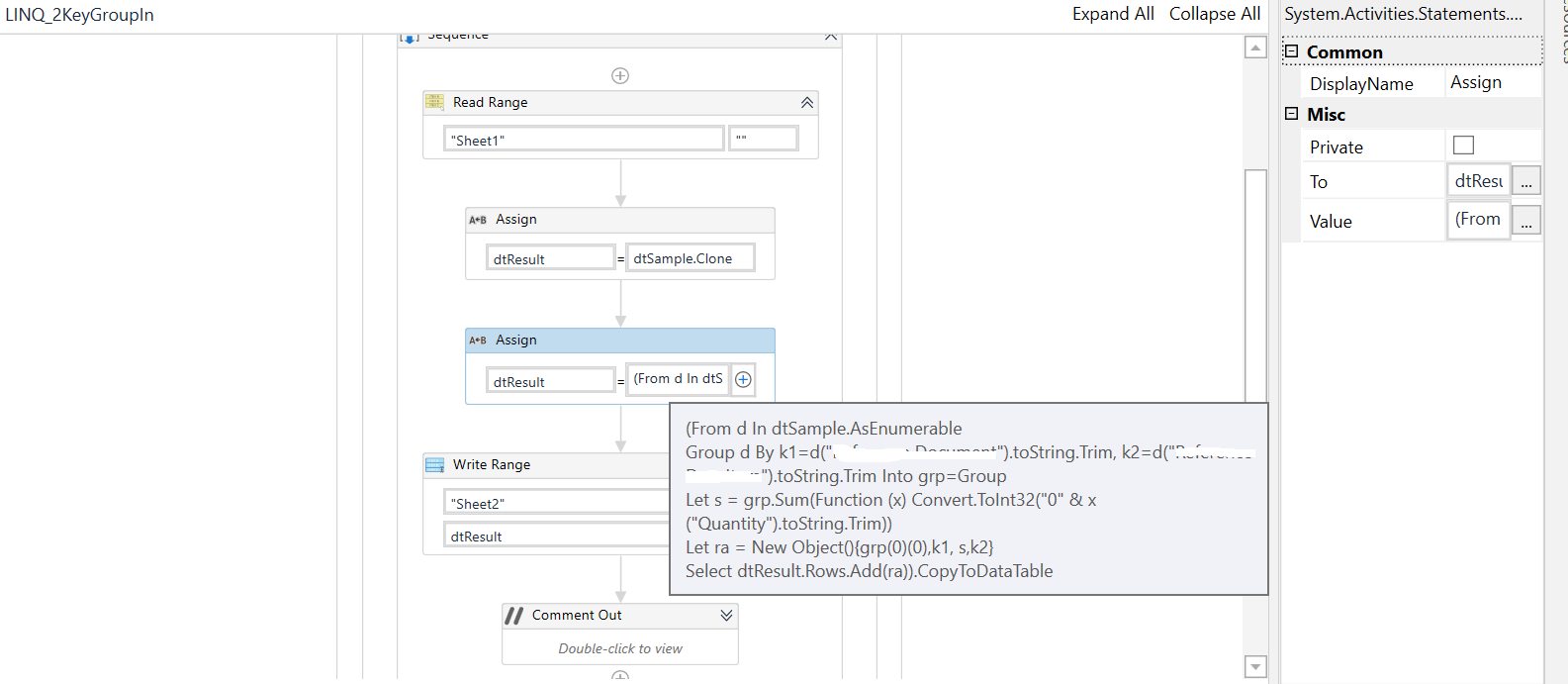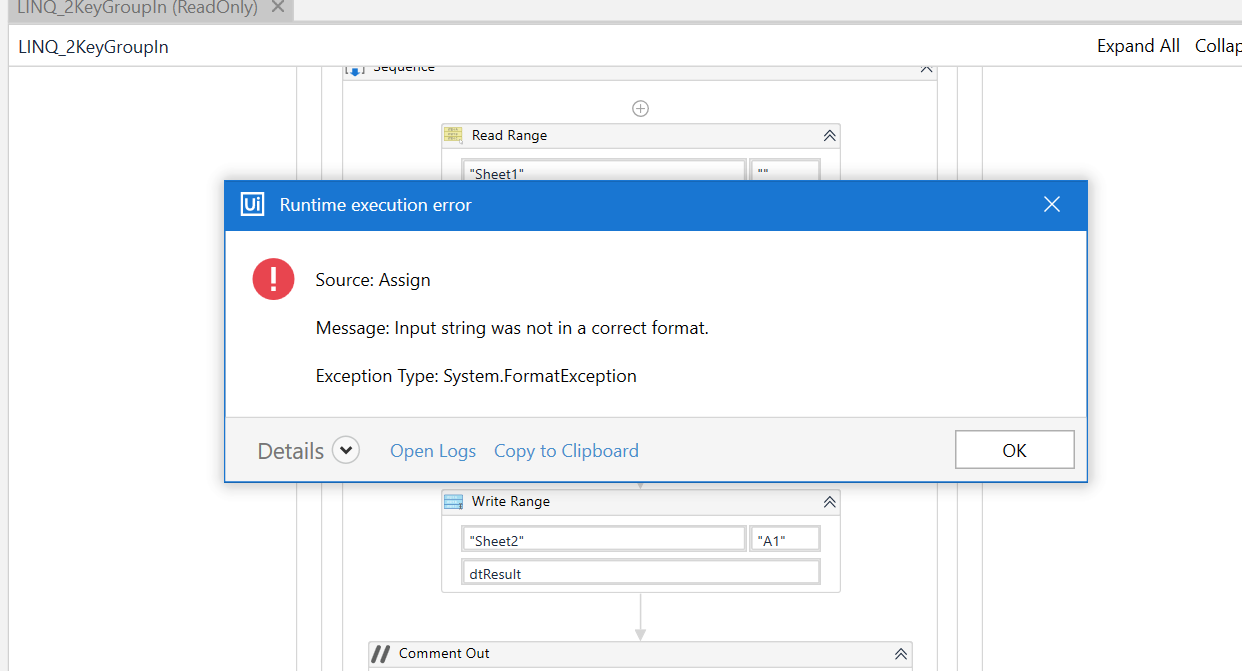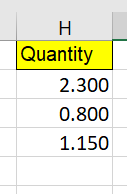# Sum column base on duplicate another column

Hi Everyone.

I have Original table as “Sheet1”… i want to check and sum “Quantity” if they same “code” value. The result received as “Sheet2”

How to do that ?

Book1.xlsx (8.8 KB)

Hi Bro @ppr

Sorry. I update again new my question.

If it same “code” and “des” columns… it will sum Quantity and delete the last row ( keep first row )

Thanks you!
Book1.xlsx (8.8 KB)

@Mr.H
give a try on:

clone / or build an empty datatable for receiving the result
e.g dtResult = YourDataTableVar.Clone

``````(From d in YourDataTableVar.AsEnumerable
Group d by k1=d("code").toString.Trim, k2=d("des").toString.Trim into grp=Group
let s = grp.Sum(function (x) Convert.ToInt32("0" & x("quantity").toString.Trim))
let ra = new Object(){grp(0)(0),k1, s,k2}
``````
2 Likes

hi bro @ppr

Is there not correct point in my workflow ?show us some values from the Quantity column. It looks like the format is different from the expected one

1 Like

Pls kindly check!give a try on following line:

``````let s = grp.Sum(function (x) Convert.ToDouble("0" & x("quantity").toString.Trim))
``````
1 Like

Hi Bro

Before i have used your solution ( another topic ) as below… but it only write duplicate row, not write another row.

In my case, i want to write all of row… and with duplicate rows ( 2 column same value ) it will check and sum value at Quantity column

@Mr.H
as mentioned it looks like some issues on the Convert.ToDouble as it get not convertable values.

``````let s = grp.Sum(function (x) Convert.ToDouble("0" & x("quantity").toString.Trim))
``````

also check while debugging the the content of the datatable after readin from excel. Another often issues are empty rows. So maybe a filtering and removing the empty rows can help as well

2 Likes

Hi Bro.

I have sent to you my file… my file only has 3 row, does not has blank row.

Thanks you!

1 Like

hi @Mr.H

for this kind of scenario first you need to filter datatable , for that filtered datatable you need to perform operation with respect to column names and add this values to another dt .

use filtered datatable activity for filtering , to perform sum operation you can use below query Cint(Dt1.AsEnumerable.Sum(Function(x)Convert.ToDecimal(x(“Columnname”))).ToString)

1 Like

Thanks you Bro!

Sorry Bro.

I have edit something and fix again my data.

(From d In dtSample.AsEnumerable
Group d By k1=d(“xxx”).toString.Trim, k2=d(“xxx”).toString.Trim Into grp=Group
Let s = grp.Sum(Function (x) Convert.ToDouble(“0” & x(“Quantity”).toString.Trim))
Let ra = New Object(){grp(0)(0),grp(0)(1), grp(0)(2), grp(0)(3), grp(0)(4), grp(0)(5), grp(0)(6), s, grp(0)(8), k1, k2}